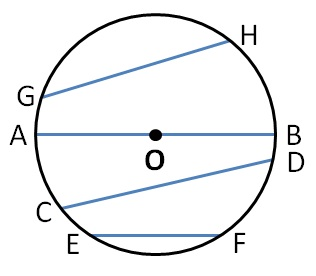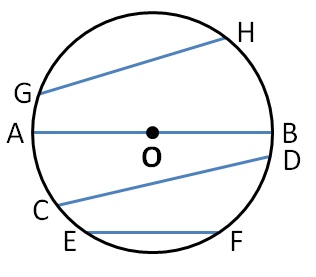Circles

Chapter 4 Class 6 Basic Geometrical Ideas
Concept wiseLine joining any two points of the circle  is the chord of the circle.

Here,

(AB) , (CD) ,  (EF) ,  (GH)  are chords of the circle.What if a chord passes through center of circle?

A chord through center of circle is the diameter of the circle.

And diameter is the longest chord.

Here, (AB) is the diameter of the circle

Learn in your speed, with individual attention - Teachoo Maths 1-on-1 Class